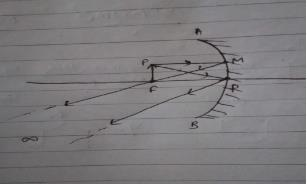Courses

# Test: Reflection By Spherical Mirrors

## 10 Questions MCQ Test Physics Class 12 | Test: Reflection By Spherical Mirrors

Description
This mock test of Test: Reflection By Spherical Mirrors for JEE helps you for every JEE entrance exam. This contains 10 Multiple Choice Questions for JEE Test: Reflection By Spherical Mirrors (mcq) to study with solutions a complete question bank. The solved questions answers in this Test: Reflection By Spherical Mirrors quiz give you a good mix of easy questions and tough questions. JEE students definitely take this Test: Reflection By Spherical Mirrors exercise for a better result in the exam. You can find other Test: Reflection By Spherical Mirrors extra questions, long questions & short questions for JEE on EduRev as well by searching above.
QUESTION: 1

### A convex and a concave mirror of radii 10 cm each are facing each other and 15 cm apart. A point object is placed midway between them. Then position of the final image if the reflection first takes place at the concave mirror and then in the convex mirror is

Solution:

for concave mirror u=−7.5 ; f=−5
(1/v)+1/u=1/f
1/v-2/15=-1/5
i.e, image formed at pole of convex mirror for convex mirror, u=0;f=+5
(1/v)+1/u=1/f
(1/v)+1/0=1/5
V=0
So the final image is formed on a pole of a convex mirror.

QUESTION: 2

### When the object is located at the F of a concave mirror then

Solution:When the object is placed at principal focus (F) of a concave mirror, a highly enlarged image is formed at infinity.

QUESTION: 3

### The focal length of a convex lens (refractive index = 1.5) in air is 20 cm. When immersed in water (refractive index = 1.33), its focal length will be​

Solution:

Focal length in air = 20 cm
Refractive index of air-water n₁= 1.33
Refractive index of air - glass n₂= 1.5
For focal length in air,
Using formula of lens
1/fair={(n2/n1)-1}(1/R1)-(1/R2)
Put the value into the formula
1/20={(1.5/1)-1}{(1/R1)-(1/R2)}
1/20=0.5{(1/R1)-(1/R2)}…1
We need to calculate the focal length in water
Using formula of lens
1/fwater={(1.5/1.33)-1}{(1/R1)-(1/R2)}
1/fwater=0.128{(1/R1)-(1/R2)}….2
fwater/20=0.5/0.128
fwater=78.125cm

QUESTION: 4

In Concave Mirror

Solution:

If a hollow sphere is cut into parts and the outer surface of the cut part is painted. Then it becomes a mirror with its inner surface as the reflecting surface This kind of mirror is known as concave mirror. Light converges at a point when it strikes and reflects back from the reflecting surface of concave mirror. Hence, it is also known as converging mirror.  When concave mirror is placed very close to the object, a magnified and virtual image is obtained. But if we increase the distance between the object and the mirror then the size of the image reduces and a real image is formed. So the image formed by concave mirror can be small or large and it can also be real or virtual.

QUESTION: 5

In mirrors how can we differentiate real image from the virtual image

Solution:

A real image is defined as one that is formed when rays of light are directed in a fixed point.  A real image can be projected or seen on a screen. The best example of a real image is the one formed on a cinema screen.
A virtual image is defined as the opposite of a real image, therefore an image that cannot be obtained on a screen is referred to as a virtual image. The explanation for this is the fact that the rays of light that form a virtual image never converge therefore a virtual image can never be projected onto a screen. The best example of a virtual image is your reflection in the mirror.
Real images are produced by intersecting rays while virtual images are produced by diverging rays.
Real images can be projected on a screen while virtual ones cannot.
Real images are formed by two opposite lenses, concave and convex.
Virtual images are always upright while real images are always inverted.

QUESTION: 6

______ mirror is called as diverging mirror

Solution:

A convex mirror is sometimes referred to as a diverging mirror due to the fact that incident light originates from the same point and will reflect off the mirror surface and diverge. The diagram at the right shows four incident rays originating from a point and incident towards a convex mirror.

QUESTION: 7

Newtonian reflecting type telescope uses

Solution:

A reflecting telescope (also called a reflector) is a telescope that uses a single or a combination of curved mirrors that reflect light and form an image. The reflecting telescope was invented in the 17th century, by Isaac Newton, as an alternative to the refracting telescope which, at that time, was a design that suffered from severe chromatic aberration. Although reflecting telescopes produce other types of optical aberrations, it is a design that allows for very large diameter objectives.
Astronomical (reflecting) telescopes. In a reflecting telescope, instead of a convex objective lens, a concave mirror is used to collect parallel rays from the object and form an image at the focal point. Then the convex eyepiece lens is used to magnify this image for the viewer.

QUESTION: 8

A convex lens has a focal length of 10cm. What is the power of the lens?

Solution:

The power of a lens is the reciprocal of the focal length with measurement in metres. The unit is diopter.

It is given that the focal length of a convex lens is 10 cm = 0.1 m.

⇒ The power of the lens is 1/0.1= 10 diopter.

QUESTION: 9

In a concave mirror when the object is located beyond C the magnification is

Solution:

In a concave mirror when the object is located beyond C the magnification is less than one as the image formed is smaller than the object.

QUESTION: 10

An image is upright and reduced in size. Which mirror is used to form such an image?

Solution:

Only Convex Mirror can form an image which is upright and reduced in size.